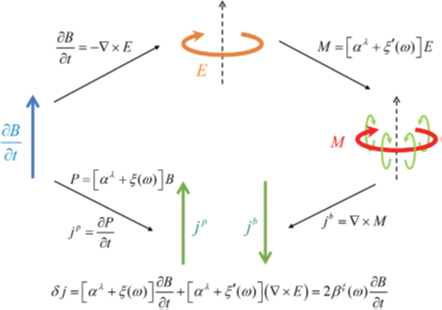# 谢心澄课题组及合作者在反对称磁电耦合相关研究中取得新进展

jp = t P = ( t α )B + α( t B )

jb = × M = ( × α ) ⋅ E + α( × E )

j = jp + jb = ( t α )B + ( × α ) ⋅ E

P = (α + β) B

M = (α – β) E

j = jp + jb = t [ α + β(ω) ]B + { × [ α - β(ω) ] } ⋅ E + 2β(ω)t B(图中包括 (1) 极化电流jp = t PP为磁电耦合诱导的电极化强度; (2)法拉第定律t B = - × E; (3)磁电耦合诱导的磁化强度M; (4) 束缚电流jb = × M。考虑新型反对称磁电耦合后，时变磁场会诱导出动态磁电流δj = 2βξ(ω)∂t Bβξ(ω) = [ ξ(ω) - ξ’(ω)] / 2。)

2022年6月10日，相关研究成果以“新型含时反对称磁电耦合”（New Type of Anticommutative Dynamical Magnetoelectric Response）为题在线发表于《物理评论快报》（Physical Review Letters）。王茂原为第一作者与通讯作者，刘海文和谢心澄为共同作者。